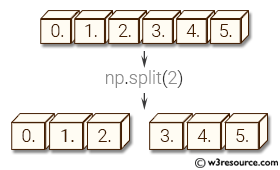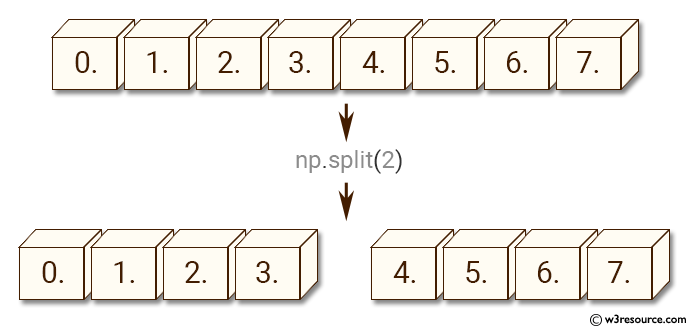# NumPy Array manipulation: split() function

## numpy.split() function

The split() function is used assemble an nd-array from given nested lists of splits.

Syntax:

`numpy.split(ary, indices_or_sections, axis=0)`Version: 1.15.0

Parameter:

Name Description Required /
Optional
ary Array to be divided into sub-arrays. Required
indices_or_sections If indices_or_sections is an integer, N, the array will be divided into N equal arrays along axis. If such a split is not possible, an error is raised.
If indices_or_sections is a 1-D array of sorted integers, the entries indicate where along axis the array is split. For example, [2, 3] would, for axis=0, result in
• ary[:2]
• ary[2:3]
• ary[3:]
If an index exceeds the dimension of the array along axis, an empty sub-array is returned correspondingly.
Required
axis The axis along which to split, default is 0. Optional

Return value:

sub-arrays : list of ndarrays A list of sub-arrays.

Raises: ValueError - If indices_or_sections is given as an integer, but a split does not result in equal division.

Example-1: numpy.split() function

``````>>> import numpy as np
>>> a = np.arange(8.0)
>>> np.split(a, 2)
[array([ 0.,  1.,  2.,  3.]), array([ 4.,  5.,  6.,  7.])]
``````

Pictorial Presentation:Example-2: numpy.split() function

``````>>> import numpy as np
>>> a = np.arange(6.0)
>>> np.split(a, [4, 5, 6, 7])
[array([ 0.,  1.,  2.,  3.]), array([ 4.]), array([ 5.]), array([], dtype=float64), array([], dtype=float64)]
``````

Python - NumPy Code Editor:

Previous: block()
Next: array_split()

﻿